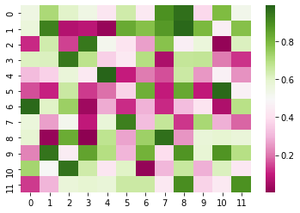Open In App
Related Articles
• Write an Interview Experience

# ColorMaps in Seaborn HeatMaps

Colormaps are used to visualize heatmaps effectively and easily. One might use different sorts of colormaps for different kinds of heatmaps. In this article, we will look at how to use colormaps while working with seaborn heatmaps.

Sequential Colormaps: We use sequential colormaps when the data values(numeric) goes from high to low and only one of them is important for the analysis.

Example of sequential colormaps:

```sns.palplot(sns.color_palette("Greens",12))
```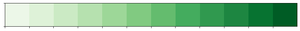Sequential color palette

```sns.palplot(sns.color_palette("Blues",12))
```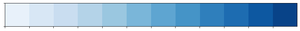Sequential color palette

Note that we have used sns.color_palette() to construct a colormap and sns.palplot() to display the colors present in the colormap. The following example shows how to implement a sequential colormap on a seaborn heatmap.

Example:

## Python3

 `import` `seaborn as sns``import` `numpy as np`` ` ` ` `np.random.seed(``0``)`` ` `# generates random values``data ``=` `np.random.rand(``12``, ``12``)`` ` `# creating a colormap``colormap ``=` `sns.color_palette(``"Greens"``)`` ` `# creating a heatmap using the colormap``ax ``=` `sns.heatmap(data, cmap``=``colormap)`

Output: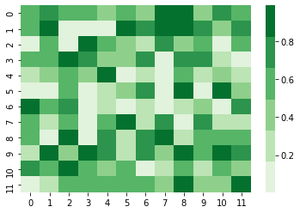Heatmap with a sequential colormap

Since “Greens” is an inbuilt colormap in seaborn, can also directly pass “Greens” to the cmap argument:

## Python3

 `import` `seaborn as sns``import` `numpy as np`` ` ` ` `np.random.seed(``0``)`` ` `data ``=` `np.random.rand(``12``, ``12``)``ax ``=` `sns.heatmap(data, cmap``=``"Greens"``)`

Output: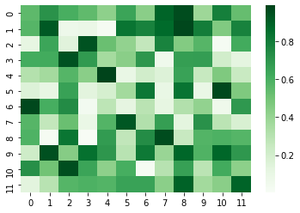Heatmap with a sequential colormap

Note that our colormap now has a continuous color intensity unlike the one before which had a discrete intensity of green for a range of values. Here is a closer look at both of the colormaps generated in the above-mentioned heatmaps: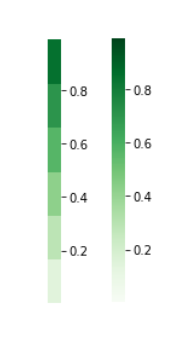Discrete(left) and Continuous(right) Colormaps

Diverging Colormaps: They are used to represent numeric values that go from high to low(and vice-versa), and both high and low values are of interest.

Here are some diverging colormaps present in seaborn:

```sns.palplot(sns.color_palette("PiYG", 12))
```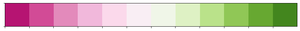Diverging color palette

```sns.palplot(sns.color_palette("coolwarm", 12))
```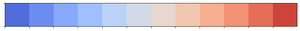Example: The following example shows how to implement a diverging colormap on a seaborn heatmap.

## Python3

 `import` `seaborn as sns``import` `numpy as np`` ` ` ` `np.random.seed(``0``)`` ` `data ``=` `np.random.rand(``12``, ``12``)``ax ``=` `sns.heatmap(data, cmap``=``"PiYG"``)`

Output: